# Estimating Watermarking Capacity in Gray Scale Images Based on Image Complexity

## Abstract

Capacity is one of the most important parameters in image watermarking. Different works have been done on this subject with different assumptions on image and communication channel. However, there is not a global agreement to estimate watermarking capacity. In this paper, we suggest a method to find the capacity of images based on their complexities. We propose a new method to estimate image complexity based on the concept of Region Of Interest (ROI). Our experiments on 2000 images showed that the proposed measure has the best adoption with watermarking capacity in comparison with other complexity measures. In addition, we propose a new method to calculate capacity using proposed image complexity measure. Our proposed capacity estimation method shows better robustness and image quality in comparison with recent works in this field.

## 1. Introduction

Determining the capacity of watermark in a digital image means finding how much information can be hidden in image without perceptible distortion, while maintaining watermark robustness against usual signal processing manipulation and attacks. Knowing the watermark capacity of an image is useful to select a watermark with a size near the capacity or in order to improve the robustness, we can repeat embedding a smaller size watermark until reaching the capacity. Usually capacity is expressed in bits per pixel (bpp) unit which is the mean capacity of image pixels for watermark embedding. Image quality assessment measures like PSNR (Peak Signal to Noise Ratio), SSIM (Structural Similarity Index Measure), and JND (Just Noticeable Difference) are used for estimating quality degradation after watermark embedding. One of the most popular measures for watermark robustness is Bit Error Rate (BER), which is the percentage of error bits in extracted watermark.

However, calculating watermark capacity in images is a complex problem, because it is influenced by many factors. Generally, there are three parameters in watermarking that have the most important role: capacity, quality, and robustness. These parameters are not independent and have side effect on each other. For example, increasing the watermark robustness by repeating the watermark bits decreases the image quality, or enhancement in quality is achieved by decreasing the capacity and vice versa.

Recently, some works for calculating watermark capacity are reported in the literature. Moulin used the concept of information hiding to calculate the capacity by considering watermarking as an information channel between transmitter and receiver [1, 2]. Barni et al. in [3, 4] introduced methods for capacity estimation based on DCT. Voloshynovisky introduced Noise Visibility Function (NVF) which estimates the allowable invisible distortion in each pixel according to its neighbor's values [5, 6]. Zhang et al. in  and authors in [10, 11] showed how to use heuristic methods to determine the capacity. In addition, some works are reported in coding system and codebooks to reduce distortion in the watermarked image [12, 13]. We shall note that these methods use different approaches to find the capacity, and the estimated capacity values have a diverged range from 0.002 bpp (bits per pixel) to 1.3 bpp .

Some approaches pay more attention to model the communication channel and attacks than the image content. We cannot neglect the fact that image content, represented here by the term image complexity, plays a very important role in capacity. This encouraged us to find the relation between complexity (image content) and capacity. This relation will help us to understand the role of image content in capacity estimation and can provide new aspects in watermarking capacity beyond the limitation of information theory which generally focuses on communication channel.

In this paper, we analyzed the relation between image complexity and watermarking capacity. In this regard, we studied the most important existing measures for image complexity and found the relation between capacity and complexity. In addition, we proposed a new complexity measure based on Region Of Interest (ROI) concept. Experimental results show that our proposed method gives better capacity estimation according to image quality degradation and watermark robustness.

The rest of paper is organized as follows. In Section 2, we discuss about complexity measures and the existing methods in image complexity and introduce a new complexity measure based on the ROI concept. In Section 3, we show how to find the best measure for complexity estimation according to quality degradation in watermarking. Section 4 is dedicated to calculate the watermark capacity based on image complexity. Finally conclusion is presented in Section 5.

## 2. Complexity Measures

There are a wide variety of definitions for image complexity depending on its application. For example, in , image complexity is related to the number of objects and segments in image. Some works have related image complexity to entropy of image intensity . In , complexity has been considered as a subjective characteristic that is represented by a fuzzy interpretation of edges in an image. In addition, there are some new definitions of image complexity but these approaches are highly application dependent [17, 18].

These definitions clarify that there are different approaches for calculating image complexity depending on the application. Since each definition, based on either subjective or objective characteristics of the input image, uses a distinct measurement or calculation algorithm, therefore, there is not any agreement on image complexity definition.

Although there is not a unique method for image complexity calculation, but there is a global agreement in classifying images by complexity. Figure 1 shows nine images with different complexities or details. These images are used in our experiments.

In the next section, we describe briefly four measures for calculating image complexity: Image Compositional Complexity (ICC) and Fractal Dimension (FD) that are used in general applications in image processing and Quad Tree method. In addition we introduced a new complexity measure named ROI that showed to be more reliable measure to estimate the watermarking capacity.

### 2.1. Image Compositional Complexity (ICC)

This measure is fully described in . In this method, a complexity measure is defined as Jensen-Shannon divergence, which expresses the image compositional complexity (ICC) of an image. This measure can be interpreted as the spatial heterogeneity of an image from a given partition. The Jensen-Shannon divergence applied to an image is given by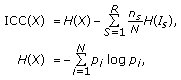(1)

where X is the original image, R is the number of segments, n s is the number of pixels in segment s, I s is a random variable associated with segment s and represents the histogram of intensities in segment s, N is the number of total pixels in image, and H is the entropy function.

The segmentation phase has an important role in this method. Thus, given an image segment, we can express the heterogeneity of an image using the JS-divergence applied to the probability distribution of each segment. For comparison with other methods, ICC values are normalized in (0, 1).

We have introduced this measure in our previous work . Briefly, quad tree representation is introduced for binary images but it can be obtained for gray scale images, too. For a gray scale image, we use the intensity variance in blocks as a measure of contrast. If the variance is lower than a predefined threshold, it means that there is not much detail in that block (i.e., pixels of the block are very similar to each other), thus, that block is not divided further. Otherwise, the division of that block into 4 blocks is continued until either a block cannot be divided any more or reaching to a block size of one pixel.

Assume thatis the level number in a quad tree withlevels, and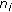is the number of nodes in level i, then we define the complexity as follow: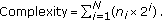(2)

The complexity values are normalized in (0, 1).

### 2.3. Fractal Dimension

Fractal Dimension (FD) is one of the texture analysis tools that show the roughness of a signal. Fractal dimension has been used to obtain shape information and distinguish between smooth (small FD) and sharp (large FD) regions [20, 21]. In , it is proposed to characterize local complexity in subimages with FD. To compute FD for images, we used the famous box-counting method .

Because there are different segments and regions in each image with different complexities, we partitioned each image into 16 equal subimages. The reason for selecting 16 subimages will be described in Sections 2–4. After that we calculated FD for all 16 subimages, and the mean value of FDs in all subimages is taken as a complexity measure for the image. Like other methods, the value of FD is normalized in (0, 1).

### 2.4. Region of Interest Method

One of the interesting subjects in image processing field is finding the regions of an image that attracts human attention more than other regions. This is the subject of Region of Interest (ROI) detection in images. Usually an image is divided into equal size blocks, a block is considered to be a region, and an ROI score is calculated for each block representing the level of interest that a human eye could have to that region.

We suggest the idea of finding the block scores according to ROI measure and then estimate the image complexity based on the total scores of blocks. To find the score of ROI in image blocks, we used the ideas suggested by Osberger in . A brief version of our work is presented in .

In summary, to find the ROI score of subimages, we calculate the following five influencing parameters to estimate the block scores corresponding to theirs ROI attractiveness. We refer to these parameters as ROI score parameters.

Intensity. The blocks of image which are closer to mid intensity of image are the most sensitive to the human eye.

Contrast. A block which has high level of contrast, with respect to its surrounding blocks, attracts the human attention and is perceptually more important.

Location. The central-quarter of an image is perceptually more important than other areas.

Edginess. A block which contains prominent edges captures the human attention.

Texture. Flat regions have not attractiveness for human eyes. Therefore, we concentrate on textured areas.

In order to determine ROI, we divide the host image into—we will discuss about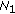and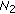later—subimages (blocks) and compute a quantitative measure (M) for each one of the five ROI score parameters at each block. The mathematical equations that we proposed to find the quantitative measure for these parameters are described below.

Intensity Metric

The mid intensity importance MIntensity of a sub image S i is computed as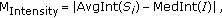(3)

where AvgInt(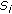) is the average luminance of sub imageand MedInt(I) is the average luminance of the whole image I.

Contrast Metric

A subimage which has the highest level of contrast with respect to its surrounding subimages attracts the human eye's attention and perceptually it is more important. If AvgInt(S i ) is the average luminance of sub imageand AvgInt() is the average luminance of all its surrounding subimages, then the contrast measure can be defined as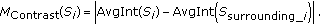(4)

Location Metric

The location importance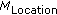of each sub image is measured by computing the ratio of number of pixels in sub image that are lying in the center-quarter of the image to the total number of pixels in the sub image. This is because eye tracking experiments have shown that viewer's eyes are directed at the center 25% of screen for viewing materials . This can be expressed as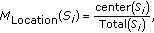(5)

where centre(S i ) is the number of pixels of sub image i lying in central quarter of the sub image and Total(S i ) is the total number of pixels of sub image, that is, the area of sub image. This parameter has an important role in ROI detection but it is not too useful for comparison between two images. The reason is that in any image, some pixels of blocks would be in quarter center and this parameter would be equal in two images. However, we consider this parameter for consistency of the proposed method with ROI detection.

Edginess Metric

The edginess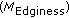is the total number of edge pixels in the sub image. We used Canny edge detection method with threshold 0.7. Using this threshold means that minor edges which usually occur in background have not any effect on edginess metric.

Texture Metric

The texture parameter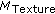is computed by variance of pixel values in each sub image. Of course there are more advanced methods to analyze textures, like Laws filter , or Tamura measures , but these methods need more computational time. It must be noted that our aim is not to classify textures but only estimate textured areas and distinguish them from flat regions. For this purpose, it has been shown thatis an appropriate measure . So, a high variance value indicates that the sub image is not flat. This measure can be calculated as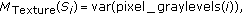(6)

where pixel_graylevels(i) is the gray level values of pixels in sub image i.

After performing the above computations for subimages, we assign a measure for each of the five ROI score parameters. The measure for each parameter is normalized in the range (0, 1). We name these normalized values as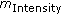,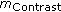,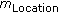,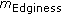, and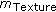, respectively.

After normalization, we must combine these five factors for each sub image to produce an overall Importance Measure (IM) for each sub image.

Although many factors which influence visual attention have been identified, little quantitative data exists regarding the exact weighting of different factors and their relationship. In addition, this relation is likely to be changed from one image to the other. Therefore, we choose to treat each factor as having equal importance. However, if it was known that a particular factor had a higher importance, a suitable weight could be easily incorporated .

To highlight the importance of regions having higher ranks according to some of the ROI score parameters, we introduced (7) in which each parameter is squared. The reason is that a simple averaging of the ROI scores will not keep the importance of highly ranked regions. We have therefore chosen to square and sum the scores to produce the final IM for each sub image S i as described by the following equation: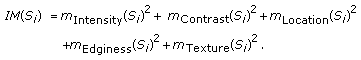(7)

The calculatedvalues for all subimages are sorted and the sub image having the highest value of IM is selected as the perceptually most important region. We divided the input image into 16 equal size subimages (blocks) and calculated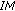for each block in order to rank them. These rankings are shown in Figure 2 for two images, Couple and Lena. (Note that only the first 8 highest score blocks are shown by numbers 18 on upper left corner of blocks).

Finally, for calculating the complexity of an image, we sum IM(S i ) of 16 blocks. The reason for choosing 16 blocks is that we assume there are not more than 16 interesting regions in natural images. However, we calculated the ROI scores while 9() or 24(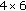) blocks were selected for each image too. But the IM values were very close to the scores calculated with 16 blocks (i.e., about %7 tolerance).

After calculation of ROI score in each sub image, as a rule of thumb, we find out that the mean of all sub image scores will give a good estimation for image complexity. This means that images with high contrast, edginess, and texture could be considered as images with high complexity. Table 1 shows the result of complexity for 9 standard images of Figure 1, calculated by the four above mentioned complexity methods (ICC, Quad tree, Fractal Dimension, and ROI). All complexity measures are normalized in (0, 1).

## 3. Image Complexity and Quality Degradation

In this section, we use three famous watermarking algorithms which work in different domains for finding the relation between complexity measures and watermarking artifacts on images. These algorithms are amplitude modulation  in spatial domain, Cox method in DCT domain , and Kundur algorithm in wavelet domain . For simplicity, we will refer to these algorithms as Spatial, DCT, and Wavelet in the rest of this paper.

We use 2000 images with different resolutions and sizes from the Corel database and calculated the complexity measure of each image using the four measures discussed in Section 2. Then we watermark each image using three mentioned watermarking methods (Spatial, DCT, and Wavelet). To compare the visual quality of host image and watermarked image, we use the SSIM (Structural Similarity Index Measure)  and Watson JND (Just Noticeable Difference)  measures that are two state-of-the-art image quality assessment measures. These measures consider the structural similarity between images as human visual system and provide better results compared to the traditional methods such as PSNR (Peak Signal to Noise Ratio) .

In our experiment, we use a watermark pattern with 256 bits (a usual watermark size) in all three watermarking methods. To compare the results, Figures 310 show the relation between each complexity measure and the visual quality degradation measures (SSIM and JND) averaged on 2000 images mentioned before.

However, we must emphasis that in this section no watermarked image is degraded by any manipulation (attacks). Therefore, we can extract all bits of watermark without error, which means that the Bit Error Rate (BER) is zero. We will discuss about the robustness of proposed method in more detail in Section 4.

The relation between image quality degradation and complexity after watermark embedding is shown in Figures 310 using the four different complexity measures.

In the following we describe the results of Figures 310 in detail.

1. (a)

In Figures 310, a simple relation between image complexity and visual quality can be understood. That is, when complexity of an image is higher, then the visual quality of watermarked image is higher too (e.g. higher SSIM or JND). This shows that complex images have higher capacity for watermarking.

2. (b)

In ICC, Quad tree and Fractal dimension methods (Figures 3, 4, 5, 7, 8, and 9), there are some irregularities or nonlinear relation between complexity and visual quality measures, but the ROI measure can give better estimation on capacity because as seen in Figures 6 and 10, the curves have a straight linear shape. For better comparison of different methods, we calculate the correlation coefficient between each complexity measures and quality degradation in different watermarking methods (achieved from 2000 images). The result is presented in Table 2. As it is seen the correlation coefficient of ROI method is much better than other measures. This means that the ROI complexity measure has a very close to linear relation with watermarking capacity as its correlation coefficient is 0.92. So we can estimate the quality degradation with ROI measure much better than other methods.

1. (c)

Wavelet method shows a better match with quad tree measure. This is concluded because of more regularity in its curve compared to curves related to Spatial and DCT as shown in Figure 4. This is a logical fact, because the complexity measure based on Quad tree uses similar concept of dividing an image into 4 blocks as used in multiscale watermarking methods such as wavelet.

## 4. Capacity Estimation

Finally for calculating image capacity, we consider watermarking as communication channel with side information . Briefly, in this approach, watermarking is a form of communications. The requirement that the fidelity of the media content must not be impaired implies that the magnitude of the watermark signal must be very small in comparison to the content signal, analogous to power constraint in traditional communications. This characteristic of watermark detection and considering the content (host image) as noise has led us to think of watermarking as a form of communications. But when the media content is considered as noise, no advantage is taken of the fact that the content is completely known to the watermark embedder.

Therefore, it is better to consider watermarking as an example of communication with side information. This form of communication was introduced by Shannon who was interested in calculating the capacity of a channel. Modeling watermarking as a communication with side information allows more effective watermarking algorithms to be designed and originally introduced in .

Here we used a modified version of the famous Shannon channel capacity equation (8) as used by many other researchers to estimate the watermark capacity [1, 3, 9].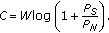(8)

Zhang and et al. in  suggested that the watermark power constraint P s should be associated with the content of an image. He introduced Maximum Watermark Image (MWI) in which the amplitude (value) of each pixel is the maximum allowable distortion calculated by Noise Visibility Function . Then the watermark capacity could be calculated by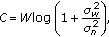(9)

where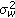is the variance of MWI and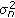is the variance of noise.is the bandwidth of channel. In an image with M pixels,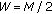according to Nyquist sampling theory . We used Equation (9) but instead ofwe used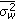as (10)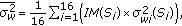(10)

where IM(S i ) is the Importance Measure as (7) andis the variance of intensity values in S i (sub image i). In other words, we calculate the average variance of 16 subimages (), as a weighted mean of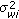where, IM(S i ) (importance measure according to ROI method) is considered as weight. Comparison of capacity results for "Lena" and "Fishing boat" are shown in Table 3.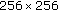Table 3 Comparison of watermarking capacity estimation in bits (images are ).

Although the capacity values estimated by Zhang are higher than our method, but in the following we show that our method gives a more precise limit for the capacity.

To compare the preciseness of capacity estimation of Zhang and our method; according to watermark robustness against noise, we watermarked 2000 images in Spatial, Wavelet, and DCT domains using 10 random watermarks with different sizes (64, 128, 256, 1024, and 2048 bits). This process provides 20,000 watermarked images. Note that in all of the watermarked images the quality is acceptable due to SSIM, JND, and PSNR. We calculated the Bit Error Rate (BER) of our proposed method by applying Gaussian noise with different variances and compared the results with that of Zhang method (as reported in ).

This comparison is presented in Figure 11. It shows that in equal watermark capacity estimated by Zhang and our proposed method, the BER of our method is always lower than Zhang method. We conclude that, the higher capacity estimation in  is too optimistic and our method gives better robustness in equal capacity. It means that our method estimates the capacity more accurately.

## 5. Conclusion

Determining the capacity of watermark in a digital image means finding how much information can be hidden in the image without perceptible distortion and acceptable watermark robustness. In this paper, we introduced a new method for calculating watermark capacity based on image complexity. Although a few researchers have studied image complexity independently, in this paper we proposed a new method for estimating image complexity based on the concept of Region Of Interest (ROI) and used it to calculate watermarking capacity. For this purpose, we analyzed the relation between watermarking capacity and different complexity measures such as ICC, Quad tree, Fractal dimension, and ROI. We calculated the degradation of images with SSIM and JND quality measures with different watermarking algorithms in spatial, wavelet and, DCT domains.

Our experimental results showed that using the ROI measure to calculate the complexity provides more accurate estimation for watermark capacity. In addition, we proposed a method to calculate the capacity in bits per pixel unit according to the complexity of images by considering image watermarking as a communication channel with side information.

The experimental results show that our capacity estimation measure improves the watermark robustness and image quality in comparison with the most recent similar works.

## References

1. 1.

Moulin P, Mihcak MK, Gen-Iu L: An information-theoretic model for image watermarking and data hiding. Proceedings of the IEEE International Conference on Image Processing, 2000 3: 667-670.

2. 2.

Cox J, Milller ML, McKellips A: Watermarking as communications with side information. Proceedings of the IEEE 1999, 87(7):1127-1141. 10.1109/5.771068

3. 3.

Barni M, Bartolini F, De Rosa A, Piva A: Capacity of the watermark channel: how many bits can be hidden within a digital image? Security and Watermarking of Multimedia Contents, 1999, Proceedings of SPIE 3657: 437-448.

4. 4.

Barni M, Bartolini F, De Rosa A, Piva A: Capacity of full frame DCT image watermarks. IEEE Transactions on Image Processing 2000., 9(8):

5. 5.

Voloshynovsky S, Herrigel A, Baum N: A stochastic approach to content adaptive digital image watermarking. Proceedings of the 3rd International Workshop on Information Hiding, 1999, Lecture Notes in Computer Science 1768: 211-236.

6. 6.

Pereira S, Voloshynoskiy S, Pun T: Optimal transform domain watermark embedding via linear programming. Signal Processing 2001, 81(6):1251-1260. 10.1016/S0165-1684(01)00042-1

7. 7.

Zhang F, Zhang H: Wavelet domain watermarking capacity analysis. Electronic Imaging and Multimedia Technology IV, February 2005, Beijing, China, Proceedings of SPIE 5637: 657-664.

8. 8.

Zhang F, Zhang H: Watermarking capacity analysis based on neural network. Proceedings of the International Symposium on Neural Networks, 2005, Chongqing, China, Lecture Notes in Computer Science

9. 9.

Zhang F, Zhang X, Zhang H: Digital image watermarking capacity and detection error rate. Pattern Recognition Letters 2007, 28(1):1-10. 10.1016/j.patrec.2006.04.020

10. 10.

Yaghmaee F, Jamzad M: Computing watermark capacity in images according to their quad tree. Proceedings of the 5th IEEE International Symposium on Signal Processing and Information Technology (ISSPIT '05), December 2005, Athens, Greece

11. 11.

Yaghmaee F, Jamzad M: Estimating data hiding capacity of gray scale images based on its bitplanes structure. Proceedings of the 4th International Conference on Intelligent Information Hiding and Multimedia Signal Processing, August 2008, Harbin, China

12. 12.

Erez U, Shamai S, Zamir R: Capacity and lattice strategies for canceling known interference. IEEE Transactions on Information Theory 2005, 51(11):3820-3833. 10.1109/TIT.2005.856935

13. 13.

Yang Y, Sun Y, Stankovi V, Xiong Z: Image data hiding based on capacity-approaching dirty-paper coding. Security, Steganography, and Watermarking of Multimedia Contents VIII, January 2006, San Jose, Calif, USA, Proceedings of SPIE 6072:

14. 14.

Peters RA, Strickland RN: Image complexity metrics for automatic target recognizers. Proceedings of the Automatic Target Recognizer System and Technology Conference, October 1990 1-17.

15. 15.

Rigau J, Feixas M, Sbert M: An information-theoretic framework for image complexity. Proceedings of the Eurographics Workshop on Computational Aesthetics in Graphics, Visualization and Imaging, 2005

16. 16.

Mario M, Chacon D, Corral S: Image complexity measure: a human criterion free approach. Proceedings of the Annual Meeting of the North American Fuzzy Information Processing Society (NAFIPS '05), June 2005 241-246.

17. 17.

Liu Q, Sung AH: Image complexity and feature mining for steganalysis of least significant bit matching steganography. Information Sciences 2008, 178(1):21-36. 10.1016/j.ins.2007.08.007

18. 18.

Cardaci M, Di Gesù V, Petrou M, Tabacchi ME: A fuzzy approach to the evaluation of image complexity. Fuzzy Sets and Systems 2009, 160(10):1474-1484. 10.1016/j.fss.2008.11.017

19. 19.

Jamzad M, Yaghmaee F: Achieving higher stability in watermarking according to image complexity. Scientia Iranica Journal 2006, 13(4):404-412.

20. 20.

Morgan S, Bouridane A: Application of shape recognition to fractal based image compression. In Proceedings of the 3rd International Workshop in Signal and Image Processing (IWSIP '96), January 1996, Manchester, UK. Elsevier Science;

21. 21.

Conci A, Aquino FR: Fractal coding based on image local fractal dimension. Computational and Applied Mathematics Journal 2005, 24(1):83-98.

22. 22.

Chen CH, Pau LF: Texture analysis. Handbook of Pattern Recognition and Computer Vision 1998, 207-248.

23. 23.

Osberger W, Maeder A: Automatic identification of perceptually important regions in image. Proceeding of the 14th IEEE Conference on Pattern Recognition, 1998

24. 24.

Yaghmaee F, Jamzad M: Estimating data hiding capacity of gray scale images based image complexity. Proceedings of the 4th International Conference on Intelligent Information Hiding and Multimedia Signal Processing, August 2008, Harbin, China

25. 25.

Elias G, Shervin G, Wise J: Eye movement while viewing NTSC format television. SMPTE Psychophysics Subcommittee white paper, March 1984

26. 26.

Laws K: Textured image segmentation, Ph.D. dissertation. University of Southern California; January 1980.

27. 27.

Tamura H, Mori S, Yamawaki T: Texture features corresponding to visual perception. IEEE Transactions on Systems, Man, and Cybernetics 1978, 8(6):460-473.

28. 28.

Mohanty S, Guturu P, Kougianos E, Pati N: A novel invisible color image watermarking scheme using image adaptive watermark creation and robust insertion-extraction. Proceedings of the 8th IEEE International Symposium on Multimedia, 2006 153-160.

29. 29.

Kutter M, Jordan F, Bossen F: Digital watermarking of color images using amplitude modulation. Journal of Electronic Imaging 1998, 7(2):326-332. 10.1117/1.482648

30. 30.

Cox J, Kilian J, Leighton T, Shamoon T: Secure spread spectrum watermarking for multimedia. IEEE Transactions on Image Processing 1997, 6(12):1673-1687. 10.1109/83.650120

31. 31.

Kundur D, Hatzinakos D: A robust digital image watermarking method using wavelet-based fusion. Proceedings of the IEEE International Conference on Image Processing, October 1997, Santa Barbara, Calif, USA 1: 544-547.

32. 32.

Wang Z, Bovik A, Sheikh H: Image quality assessment: from error visibility to structural similarity. IEEE Transaction on Image Processing 2004., 13(4):

33. 33.

Watson A: DCT quantization matrices visually optimized for individual images. Human Vision, Visual Processing, and Digital Display IV, February 1993, San Jose, Calif, USA, Proceedings of SPIE 1913: 381-392.

34. 34.

Sheikh HR, Sabir M, Bovik AC: A statistical evaluation of recent full reference image quality assessment algorithms. IEEE Transaction on Image Processing 2006., 15(11):

## Acknowledgment

This research was financially supported by Iran Telecommunication Research Center (ITRC), as a Ph.D. thesis support program.

## Author information

Authors

### Corresponding author

Correspondence to Farzin Yaghmaee.

## Rights and permissions

Reprints and Permissions

Yaghmaee, F., Jamzad, M. Estimating Watermarking Capacity in Gray Scale Images Based on Image Complexity. EURASIP J. Adv. Signal Process. 2010, 851920 (2010). https://doi.org/10.1155/2010/851920

• Revised:

• Accepted:

• Published:

### Keywords

• Fractal Dimension
• Complexity Measure
• Watermark Image
• Host Image
• Image Complexity